The cool thing about the last two formulas is that you can just jump in and find ANY term of the sequence!

For, let's find the 100th term: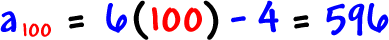Easy!

Some formulas are a bit trickier.  Remember what happened with the Fibonacci numbers?  We COULDN'T just pop out the 100th term, since each term was based on the two previous terms.

Here's the official formula for the Fibonacci's: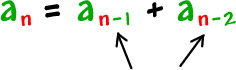These notations aren't as bad as they look!

If we want the 10th term, then n = 10...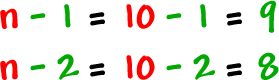So,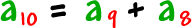Let's build the Fibonacci's using the formula:

We need to be given the first two terms to start or we can't do anything: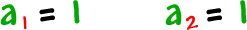let n = 3: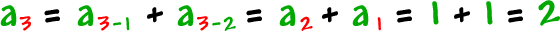let n = 4: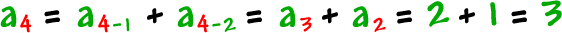let n = 5: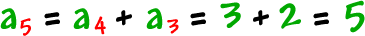let n = 6: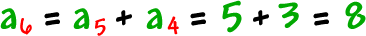and so on.